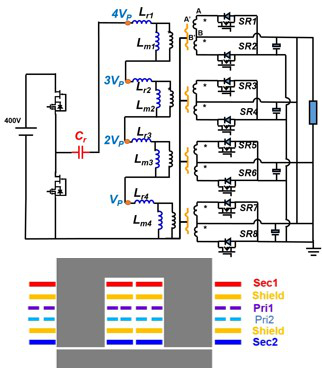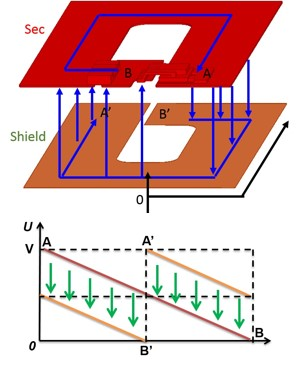LIBRARY

# Analysis and Reduction of Common Mode EMI Noise for Resonant Converters

Year: 2014Fig. 1. Matrix transformer LLC with shielding.
There are many topologies of resonant converters. The topologies can be grouped accord-ing to the number of resonant elements. The simplest topology of resonant converter is the two-element resonant converter: the series resonant converter (SRC) and the parallel resonant con-verter (PRC). Unfortunately, these topologies cannot be applied widely due to their limitations. In practice, the three-element resonant converter is the most popular topology of resonant convert-er. In the three-element resonant converter group, there are a total of 36 different topologies. Among them, the most practical topologies are LLC, CLL and LCL resonant converters because of their LLC-like gain characteristic.

The source of CM noise is the voltage pulse (dv/dt) of the switching devices. The propaga-tion path of CM noise is the parasitic capacitance, e.g. the inter-winding capacitance of the transformer, or the drain-to-ground capacitance of the switches. A high switching frequency not only brings the resonant converter high power density, but may also cause high dv/dt on the converter nodes, which may have resonant converters suffer from severe EMI issues. Different topologies of resonant converter have different dv/dt characteristics. After analyzing the dv/dt characteristic of three different topologies of resonant converter (LLC, CLL and LCL) and com-paring their CM noise performance, we find that the LCL resonant converter has the best CM noise performance among three topologies, but it is not the most popular one in commercial products. The LLC resonant converter is the most widely used topology, however it has poor CM noise performance.

A 1 MHz 400/12V 1kW LLC converter with a matrix transformer is used as an example. The peak efficiency of this LLC converter is 95.4% and the power density is 710W/in3. The typical commercial product has 98% efficiency and 60W/in3 power density. However, the matrix trans-former has very large inter-winding capacitance which leads to severe CM noise problems. Much research has been done to reduce the CM noise in LLC resonant converters. However, there is lack of study of CM noise reduction in matrix transformers. We have devised a novel shielding method to reduce the CM noise of LLC resonant converters. With shielding, the CM noise of LLC resonant converters is reduced significantly.

Loss analysis of the shielding is provided using the FEA tool. The experiment results show that the shielding introduces very little extra loss to the converter. Furthermore, a way to further re-duces the loss and improve efficiency is introduced and verified by the FEA tool.Fig. 2. Voltage distribution of shielding and Sec. winding.